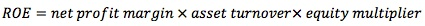Subject 3. The DuPont System
#cfa #cfa-level-1 #financial-analysis-techniques #financial-reporting-and-analysis #has-images
The breakdown of ROE into component ratios to assess the impact of those ratios is generally referred to as the DuPont Model.

• ROE = net income / common equity
• ROE = (net income / net sales) x (net sales / common equity). Therefore, ROE = (net profit margin) x (equity turnover).
• ROE = (net income / net sales) x (net sales / total assets) x (total assets / common equity)

Each of these components impacts the overall return to shareholders. An increase in profit margin, asset turnover, or leverage can all increase the return. There is a downside as well. If a company loses money in any year, the asset turnover or financial leverage multiplies this loss effect.This implies that to improve its return on equity, a company should become more:

• Profitable (increase net profit margin, e.g., pricing and expense control).
• Efficient (increase total asset turnover, e.g., efficiency of asset use).
• Leveraged (increase its financial leverage ratio).

A company's over- or underperformance on ROA is due to one or both of these causes, or "drivers."

The extended DuPont model takes the above three factors and incorporates the effect of taxes and interest based on the level of financial leverage. It takes the profit margin and backs up to see the effect of interest and taxes on the overall return to shareholders. Therefore the extended model starts with EBIT (Earnings Before Interest and Taxes) rather than net income.

• EAT = EBT (1 - t), where t is the company's average tax rate. Substituting EBT(1 - t) for EAT in the expanded ROE equation gives us ROE = (EBT / sales)(sales / assets)(assets / equity)(1 - t).
• EBT = EBIT - I, where I equals the company's total interest expense. Substituting (EBIT - I) into the ROE equation for EBT gives us ROE = [(EBIT / sales)(sales / assets) - (interest expense / assets)] (assets / equity) (1 - t).
• Restated in accounting terms:

ROE = [(operating profit margin) x (total asset turnover) - (interest expense rate)] x (financial leverage multiplier) x (tax retention rate)

High financial leverage does not always increase ROE; higher financial leverage will lead to a higher interest expense rate, which may offset the benefits of higher leverage.

This breakdown will help an analyst understand what happened to a company's ROE and why it happened.
If you want to change selection, open original toplevel document below and click on "Move attachment"